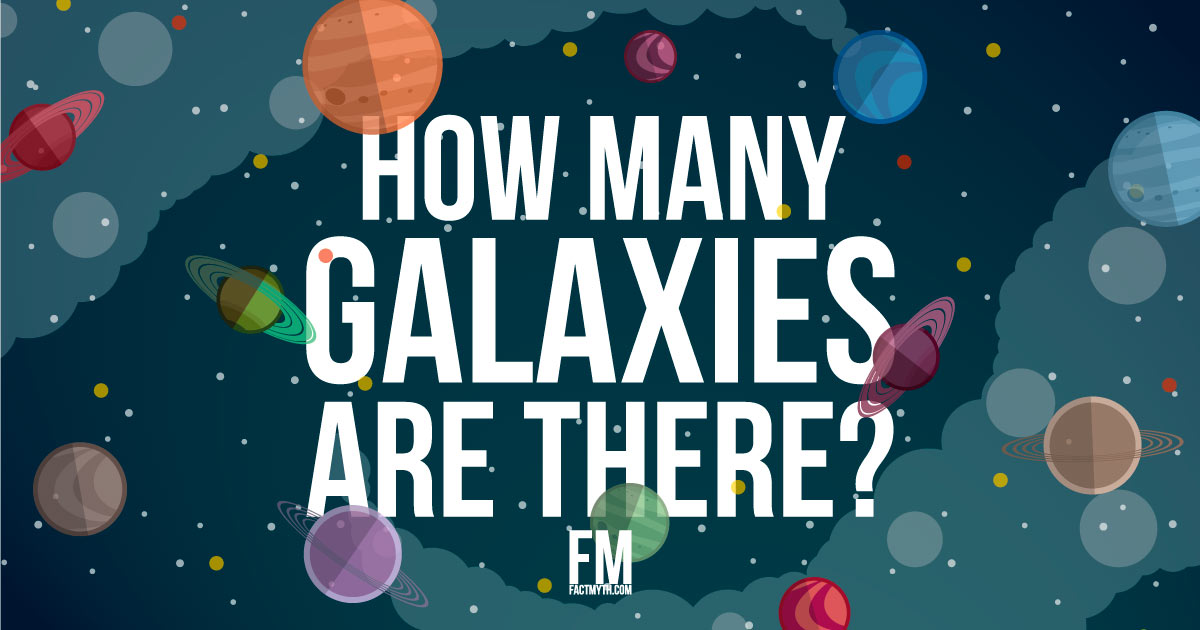Myth

We know how many galaxies, or by extension how many stars or planets, there are in the universe.

## How Many Galaxies are there in the Universe?

We don’t know exactly how many galaxies there are in the visible universe, but low estimates based on Hubble data start at 100 billion.

### How to Understand the Hubble Data

Although recent observations from Hubble currently shows there are at least 100 billion and as many as 200 billion galaxies in the observable universe.. Consider also, there is estimated to be about 100 billion stars per galaxy and 100 billion planets per galaxy. Here we are given conservative estimates based on known Hubble data, and focusing on just the visible universe, because the actual number is potentially astronomically high into the trillions in the visible universe, and it is even possible that there are an infinite number of galaxies in the visible and non-visible universe combined. We don’t currently have the technology to get a true count of galaxies, so we have to base the current count on what we reasonably know from data, and thus, we can say arrogating interpretations of the data, “While estimates among different experts vary, an acceptable range is between 100 billion and 200 billion galaxies”.

How Big Is the Universe? SciShow

Deep Universe: Hubble’s Universe Unfiltered.

FACT: Hubble images don’t use color as we would see it with our eyes, they use enhanced color based on light’s real wavelengths as seen by Hubble so we can get a true sense of what space looks like. Learn more about how Hubble images work.

FACT: When we see light from distant galaxies the age of the light is directly proportional to its distance from us. The further away an object is, the older the light. So when we observe a distant galaxy, we are seeing what the galaxy looked like billions of years ago! Stranger yet, space is expanding, so today these galaxies aren’t just billions of years older, they are further away then they were when they first sent light to us… and yes, this implies light travels in a single direction forever if not impeded (why we can see light from billions of years ago). Learn more about light.

## There are only Estimates of Galaxies or Other Celestial Objects. There are no True Counts

It’s important to understand that there is no fully accurate count of galaxies, stars, planets, or any other celestial objects in the universe. We don’t even know for sure how many celestial objects are in our solar system.

Instead, the above are very rough estimates based on past NASA data, the Hubble telescope, and other technologies. These numbers should only be used to give an idea of the vastness of the known universe, not to try to present numbers as an actual count.

What we are really saying is “we can confirm there are at least this many by all reasonable measures” and not “this is all there is in the entire visible and non-visible universe”.

How do we know how many galaxies there are in the Universe?

How Many Solar Systems in the Universe? There are estimated to be about 100 billion solar systems in the universe. 

### How Many Planets in the Universe?

There are at least 100 billion planets in our galaxy, and probably at least 1,500 planets within 50 light-years of Earth.

FACT: There is something like 100 billion times 10’s of billions solar systems in the known universe, and 100 billion times 100 billion planets.

## Why Can’t We Get an Exact Count of Celestial Objects in the Universe?

100 billion galaxies is an estimated number of galaxies based on our telescopes’ limited abilities to perceive light, and it is an estimate only of the size of the part of the universe that is currently observable.

Many have estimated higher, and given the unknown nature of the universe, this isn’t unreasonable. An exact count is likely not possible without serious advances in technology.

## What is the Observable Universe?

The visible universe refers to the part of the universe we can detect from Earth (using all available technology). The actual universe is thought to be a closed system

FACT: The estimated age of the universe is 13.73 billion years. We estimate that the diameter of the observable universe is about 28.5 gigaparsecs (93 billion light-years), putting the edge of the observable universe about 46.5 billion light-years away. The edge of the total universe is unknown, and we theorize that there may be multiple universes (multiverse). 

What Happens At The Edge Of The Universe? | Space Time | PBS Digital Studios

## Is the Universe Infinite?

There are a potentially infinite number of galaxies in the total universe or multiverse. Our estimate of the number of galaxies will be more accurate as telescopes get stronger, and our observations become more precise.

If we have infinite solar systems and infinite planets, there may be an infinite number of chances to discover life.Source: Wikipedia Commons

TIP: Infinity is a concept, not a number. It doesn’t break anything. Rather we work with infinity all the time in math and physics. We can’t calculate the circumference of a circle without the irrational number pi.

Infinite Universe?

Conclusion

We don’t know roughly how many galaxies are in the universe, but somewhere between 100 billion, to 1 trillion, to an infinite galaxies is a good guess.

1. Observable Universe contains ten times more galaxies than previously thought
2. How Many Galaxies Are There?
3. How many solar systems are in our galaxy?” Spaceplace.NASA.gov
4. About how many stars are in space?” Scienceline.UCSB.edu
5. Study Shows Our Galaxy Has at Least 100 Billion Planets” NASA.gov
6. Extra Dimensions in Space and Time” books.Google.com

"We Know How Many Galaxies There Are" is tagged with: The Universe